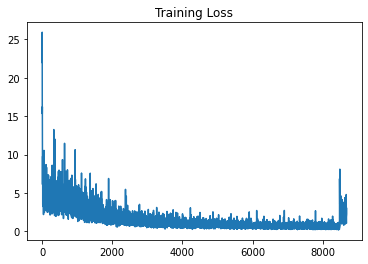# Unable to pause and resume training (loading a model) without getting a small jump in training loss

I am not able to figure out the reason for jump in training loss that I get, after loading from the saved checkpoint. I am using Adam optimizer.

Model base - Load pretrained vgg16 model weights

``````def base_model_vgg16(num_freeze_top):
vgg16 = models.vgg16(pretrained=True)
vgg_feature_extracter  = vgg16.features[:-1]

# Freeze learning of top few conv layers
for layer in vgg_feature_extracter[:num_freeze_top]:
for param in layer.parameters():

return vgg_feature_extracter.to(device)
``````

Actual Model - create new model

``````class YOLONetwork(nn.Module):
def __init__(self, extractor):
super().__init__()
self.extractor = extractor
self.conv1 = nn.Conv2d(512, 1024,3,1,1)
self.pool1 = nn.MaxPool2d(2,2)
self.conv2 = nn.Conv2d(1024, 1024,3,1,1)
self.pool2 = nn.MaxPool2d(2,2)
self.lin1 = nn.Flatten()
self.drop1 = nn.Dropout(p=0.5)
self.lin2 = nn.Linear(7*7*1024, 7*7*(num_classes + anchors_per_box*5))

def forward(self,x):
out = self.extractor(x)
out = self.pool1(F.relu(self.conv1(out)))
out = self.pool2(F.relu(self.conv2(out)))
out = self.drop1(F.relu(self.lin1(out)))
out = torch.sigmoid(self.lin2(out))

num = out.shape
return out.contiguous().view(num,7,7,-1)
``````

Creating new model and optimiser

``````extractor = base_model_vgg16(10)
net = YOLONetwork(extractor).to(device)
loss_hist = []
valid_hist = []
best_valid_loss = 100000
epoch_start = 0
``````

Saving model

``````PATH = 'drive/My Drive/saved_models/current.pt'
torch.save({
'net_state_dict': net.state_dict(),
'optimizer_state_dict': optimizer.state_dict(),
'loss_hist':loss_hist,
'valid_hist':valid_hist,
'best_valid_loss':best_valid_loss,
'epoch_start':epoch
}, PATH)
``````

``````checkpoint = torch.load(load_model, map_location=device)
loss_hist = checkpoint['loss_hist']
valid_hist = checkpoint['valid_hist']
best_valid_loss = checkpoint['best_valid_loss']
epoch_start =checkpoint['epoch_start']

net.train()
``````I am unable to figure out the reason for the training loss jump once the training is resumed from a checkpoint.

Thank you very muchI am training the network on google colab GPU. If I interrupt the training and continue it by loading saved weights, I do not see this problem.

But, if I factory reset the runtime. Reload the weights, then I can see jump in training loss.

I am also setting the same global random seed in my colab notebook.

It seems strange that one work flow seems to work on Colab, while another fails.
Are you also restoring the `optimizer.state_dict()` in both use cases?

Could you run a small test and compare the output for a fixed input, e.g. `torch.ones()`?
If you get exactly the same outputs, I would guess that the way you are restoring the data loading pipeline might create the difference.

Based on your suggestion, this is what I tried.

1. Trained the network for 1 epoch. Saved network weights after the epoch. With the existing weights (without loading it from saved checkpoint), ran the network on `torch.ones()`. (setting network to `net.train()` before running)
``````First row of output - tensor([0.5039, 0.5054, 0.4700, 0.4680, 0.3996, 0.5174, 0.5194, 0.4724, 0.4739,
0.4014, 0.4767, 0.4717, 0.4892, 0.4578, 0.4734, 0.4513, 0.4582, 0.4603],
``````
1. Loaded the network weights from saved checkpoint. Set it on `net.train()`. Ran the network on `torch.ones()`. The output that I get is
``````First row of output - tensor([0.5027, 0.5097, 0.4698, 0.4635, 0.4030, 0.5184, 0.5228, 0.4709, 0.4676,
0.4011, 0.4730, 0.4778, 0.4874, 0.4604, 0.4683, 0.4380, 0.4620, 0.4640],
``````

So, the values that I obtain as output in both these cases are different.

I take my last comment back. Since a dropout layer is used in the network, the output after net.train() will not be exactly the same.

Setting the net to `net.eval()` before running gives the same output in both cases. So, at least network weights loading is correct.

Need to check if the issue is happening due to optimizer loading or data loader change after the runtime is reset. Thanks

After spending few hours trying to figure out the problem, found the root cause. And it has nothing to do with model / optimizer loading and saving at all.

What I needed to change is

``````lab_to_val = {j:i for i,j in enumerate(training_classes)}
``````

To

``````lab_to_val = {j:i for i,j in enumerate(sorted(training_classes))}
``````

Since the label to value conversion was not happening on the sorted list, each time my runtime was reset, a single label would take different values. Ex - if `person` class takes value 1 for some run, it used to take value 4 after restarting the runtime and running the code.

After fixing this small error, I do not observe any jumps in training loss

I face the same problem. However, I don’t get your point here, where is lab_to_val = {j:i for i,j in enumerate(training_classes)}? I could not find it in your code.